## July 8, 2017

### A Bicategory of Decorated Cospans

#### Posted by John BaezMy students are trying to piece together general theory of networks, inspired by many examples. A good general theory should clarify and unify these examples. What some people call network theory, I’d just call ‘applied graph invariant theory’: they come up with a way to calculate numbers from graphs, they calculate these numbers for graphs that show up in nature, and then they try to draw conclusions about this. That’s fine as far as it goes, but there’s a lot more to network theory!

There are many kinds of networks. You can usually create big networks of a given kind by sticking together smaller networks of this kind. The networks usually do something, and the behavior of the whole is usually determined by the behavior of the parts and how the parts are stuck together.

So, we should think of networks of a given kind as morphisms in a category, or more generally elements of an algebra of some operad, and define a map sending each such network to its behavior. Then we can study this map mathematically!

All these insights (and many more) are made precise in Fong’s theory of ‘decorated cospans’:

Kenny Courser is starting to look at the next thing: how one network can turn into another. For example, a network might change over time, or we might want to simplify a complicated network somehow. If a network is morphism, a process where one network turns into another could be a ‘2-morphism’: that is, a morphism between morphisms. Just as categories have objects and morphisms, bicategories have objects, morphisms and 2-morphisms.

So, Kenny is looking at bicategories. As a first step, Kenny took Brendan’s setup and souped it up to define ‘decorated cospan bicategories’:

In this paper, he showed that these bicategories are often ‘symmetric monoidal’. This means that you can not only stick networks together end to end, you can also set them side by side or cross one over the other—and similarly for processes that turn one network into another! A symmetric monoidal bicategory is a somewhat fearsome structure, so Kenny used some clever machinery developed by Mike Shulman to get the job done:

I would love to talk about the details, but they’re a bit technical so I think I’d better talk about something more basic. Namely: what’s a decorated cospan category and what’s a decorated cospan bicategory?

First: what’s a decorated cospan? A cospan in some category $C$ is a diagram like this: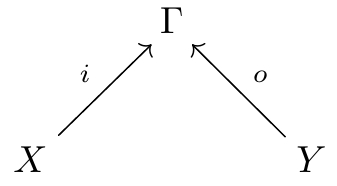where the objects and morphisms are all in $C.$ For example, if $C$ is the category of sets, we’ve got two sets $X$ and $Y$ mapped to a set $\Gamma.$

In a ‘decorated’ cospan, the object $\Gamma$ is equipped or, as we like to say, ‘decorated’ with extra structure. For example: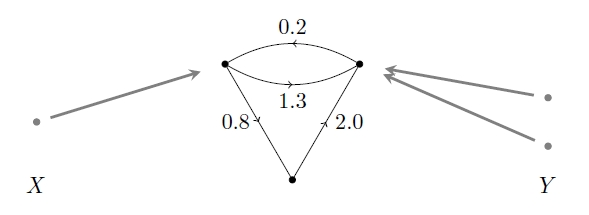Here the set $\Gamma$ consists of 3 points—but it’s decorated with a graph whose edges are labelled by numbers! You could use this to describe an electrical circuit made of resistors. The set $X$ would then be the set of ‘input terminals’, and $Y$ the set of ‘output terminals’.

In this example, and indeed in many others, there’s no serious difference between inputs and outputs. We could reflect the picture, switching the roles of $X$ and $Y,$ and the inputs would become outputs and vice versa. One reason for distinguishing them is that we can then attach the outputs of one circuit to the inputs of another and build a larger circuit. If we think of our circuit as a morphism from the input set $X$ to the output set $Y,$ this process of attaching circuits to form larger ones can be seen as composing morphisms in a category.

In other words, if we get the math set up right, we can compose a decorated cospan from $X$ to $Y$ and a decorated cospan from $Y$ to $Z$ and get a decorated cospan from $X$ to $Z.$ So with luck, we get a category with objects of $C$ as objects, and decorated cospans between these guys as morphisms!

For example, we can compose this:and this: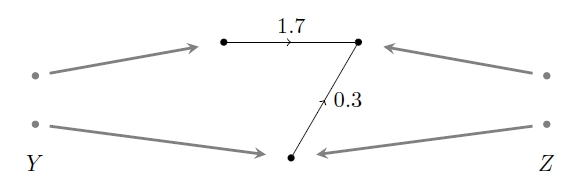to get this: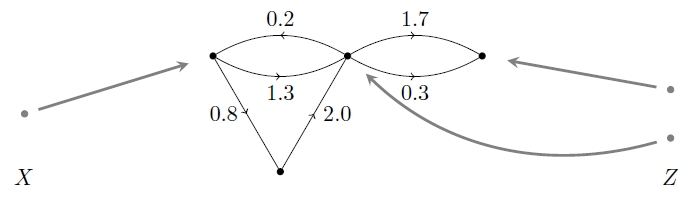What did I mean by saying ‘with luck’? Well, there’s not really any luck involved, but we need some assumptions for all this to work. Before we even get to the decorations, we need to be able to compose cospans. We can do this whenever our cospans live in a category with pushouts. In category theory, a pushout is how we glue two things together.

So, suppose our category $C$ has pushouts. IF we then have two cospans in $C,$ one from $X$ to $Y$ and one from $Y$ to $Z:$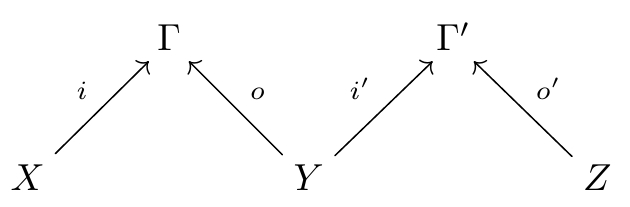we can take a pushout: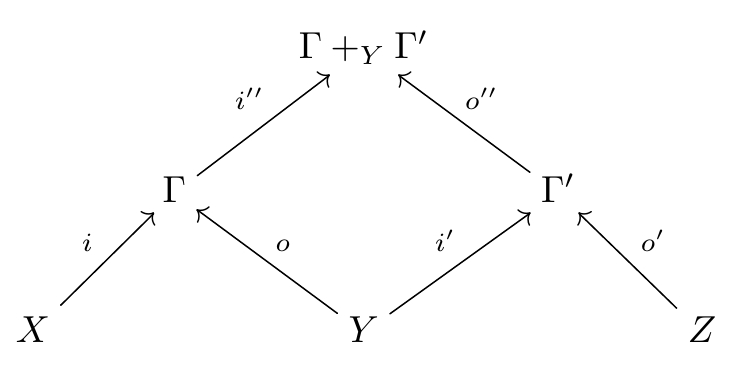and get a cospan from $X$ to $Z:$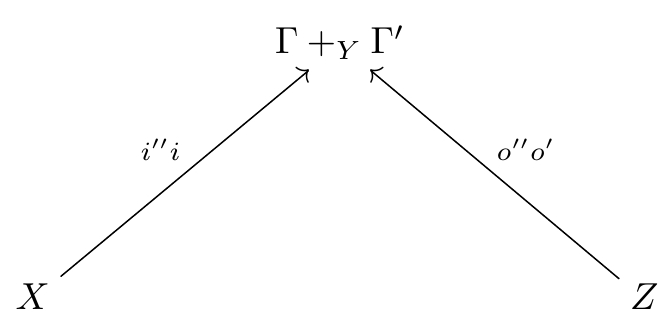All this is fine and dandy, but there’s a slight catch: the pushout is only defined up to isomorphism, so we can’t expect this process of composing cospans to be associative: it will only be associative up to isomorphism.

What does that mean? What’s an isomorphism of cospans?

I’m glad you asked. A map of cospans is a diagram like this: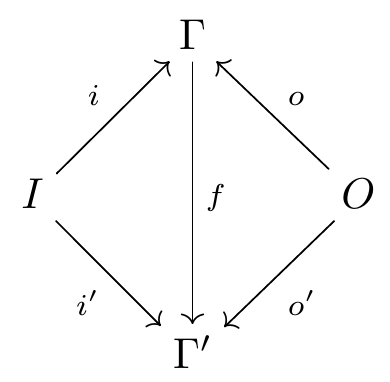where the two triangles commmute. You can see two cospans in this picture; the morphism $f$ provides the map from one to the other. If $f$ is an isomorphism, then this is an isomorphism of cospans.

To get around this problem, we can work with a category where the morphisms aren’t cospans, but isomorphism classes of cospans. That’s what Brendan did, and it’s fine for many purposes.

But back around 1972, when Bénabou was first inventing bicategories, he noticed that you could also create a bicategory with

• objects of $C$ as objects,
• spans in $C$ as morphisms, and
• maps of spans in $C$ as 2-morphisms.

Bicategories are perfectly happy for composition of 1-morphisms to be associative only up to isomorphism, so this solves the problem in a somewhat nicer way. (Taking equivalence classes of things when you don’t absolutely need to is regarded with some disdain in category theory, because it often means you’re throwing out information—and when you throw out information, you often regret it later.)

So, if you’re interested in decorated cospan categories, and you’re willing to work with bicategories, you should consider thinking about decorated cospan bicategories. And now, thanks to Kenny Courser’s work, you can!

He showed how the decorations work in the bicategorical approach: for example, he proved that whenever $C$ has finite colimits and

$F : (C,+) \to (\mathrm{Set}, \times)$

is a lax symmetric monoidal functor, you get a symmetric monoidal bicategory where a morphism is a cospan in $C:$with the object $\Gamma$ decorated by an element of $F(\Gamma).$

Proving this took some virtuosic work in category theory. The key turns out to be this glorious diagram:For the explanation, check out Proposition 4.5 in his paper.

I’ll talk more about applications of cospan bicategories when I blog about some other papers Kenny Courser and Daniel Cicala are writing.

Posted at July 8, 2017 11:59 PM UTC

TrackBack URL for this Entry:   https://golem.ph.utexas.edu/cgi-bin/MT-3.0/dxy-tb.fcgi/2970

### Re: A Bicategory of Decorated Cospans

Does “insertion” of networks also fit into this picture somehow? By that I mean that if I have a network $N$ and a node $n\in N$, and another network $M$ such that the connections of $n$ match up with the inputs/outputs of $M$, then I can make a new network in which $n$ is removed and all of $M$ is “inserted” in its place.

I don’t know what the appropriate generality is in which to define that, but recently I happened to be thinking about something similar for a different purpose, and it seemed convenient to think about it in terms of composition in a bicategory of “decorated relations” in which a network is a morphism from its “input/output type” to its “node types”. I wonder whether anyone has written down something like that before?

Posted by: Mike Shulman on July 9, 2017 12:29 PM | Permalink | Reply to this

### Re: A Bicategory of Decorated Cospans

Wouldn’t that just be inverse coarse-graining? The inverse of simplifying a network?

At least that’s what I’d assume “simplifying a network” to usually mean.

Posted by: kram1032 on July 10, 2017 2:06 PM | Permalink | Reply to this

### Re: A Bicategory of Decorated Cospans

Maybe; I’m not familiar with the phrase “simplifying a network”. (John said something about simplifying networks in his post, but I assumed it was just an informal usage.) In any case, that seems to just rephrase the question.

Posted by: Mike Shulman on July 10, 2017 9:56 PM | Permalink | Reply to this

### Re: A Bicategory of Decorated Cospans

Hi Mike!

I don’t know if your insertion of networks idea would fit into this particular framework, but it might be able to be captured using double pushout graph rewriting. I’m not an expert on this, but the idea is that we start with a monic span (meaning that both legs are monic)

$A \leftarrow B \rightarrow M$

and a morphism $B \to D$. Here, $A$ is the node $n$ together with all the nodes adjacent to $n$ as well as edges between those nodes and the node $n$. We take $B$ to be just the collection of nodes adjacent to $n$ and $M$ is the network that we want to insert in place of $n$. $D$ is the original network $N$ with the node $n$ (and obviously any edges incident with it) removed, so $D$ can be thought of as the “frame” in which we are removing $n$ and replacing it with $M$. All the morphisms are just inclusions. This gives us two adjacent cospans with a shared leg. If we take the pushout of the cospan

$A \leftarrow B \rightarrow D$

we get the original network $N$ that we started with and if we take the pushout of the other cospan

$D \leftarrow B \rightarrow M$

we get a new network that has the network $M$ in place of the node $n$.

The span $A \leftarrow B \rightarrow M$ above is an example of a graph rewrite, which says “replace $A$ by $M$ if $B$ is present”, so your condition of the inputs and outputs of $M$ matching up with those of $n$ would be captured by this span.

If $C$ is a topos so that we may push and pull as we please, Daniel Cicala has shown in Spans of cospans that monic spans like the one above can be seen as 2-morphisms in a bicategory $MonicSp(Csp(C))$, whose objects are that of $C$, morphisms are cospans in $C$ and 2-morphisms are (isomorphism classes of) monic spans of cospans in $C$. Then he and I showed that this bicategory is symmetric monoidal (compact closed, actually) in Bicategories of spans and cospans using a result of some guy named Shulman :)

Posted by: Kenny Courser on July 11, 2017 3:34 AM | Permalink | Reply to this

### Re: A Bicategory of Decorated Cospans

Ah, wonderful! Thanks so much, I think double pushout graph rewriting is exactly what I wanted. I remember now reading your paper “spans of cospans” and not really understanding section 4, and likewise not really understanding the double-pushout rewriting section of the Lack-Sobocinski paper about adhesive categories. But (as seems to happen so often) now that I have an application of my own in mind and have come halfway towards reinventing the concept myself, I can appreciate it much better.

What I really want is a nice abstract notion of the associativity and unitality of this sort of rewriting, and it seems natural to try to incorporate it into a bicategory. (This is not really germane to this post, but I hope you won’t mind my asking you questions about it here.) I think that in my situation I want to “compose” such rewrites using “dependency relations” as described in Definition 5.6 of Lack-Sobocinski, but in the special case where the morphism $t$ is an isomorphism. This suggests to me that maybe I should be looking at a bicategory constructed from some adhesive category $\mathbf{C}$ whose objects are those of $\mathbf{C}$ and whose morphisms from $X$ to $Y$ are diagrams like

$X \xleftarrow{l} K \xrightarrow{r} R \xleftarrow{s} Y$

in which $l,r$ are monic and perhaps some other condition holds. They would be composed like in Definition 5.8 of “concurrent production” in Lack-Sobocinski, with the “some other condition” ensuring that the pushout-complement $E_2'$ exists. Do you know whether anything like this exists in the literature? Lack-Sobocinski don’t mention any sort of associativity for the “concurrent production” construction.

Posted by: Mike Shulman on July 14, 2017 11:22 PM | Permalink | Reply to this

### Re: A Bicategory of Decorated Cospans

Hi Mike.

How are you composing morphisms of the form $L \leftarrow K \rightarrow R \leftarrow Y$ with the dependency relations? I don’t immediately see how one would stick another such morphism on the end of that. I can see more clearly, however, composing morphisms of the form $X \rightarrow L \leftarrow K \rightarrow R \leftarrow Y$. Then given a morphism like $Y \rightarrow L' \leftarrow K' \rightarrow R' \leftarrow Z$, and assuming enough colimits, we can pushout over $R \leftarrow Y \rightarrow L'$ which is a piece of the composition. But as you mentioned, we still need to ensure the pushout complement exists. Sorry, but I’m not aware of any sufficient conditions for this.

However here is what crossed my mind; hopefully it is helpful for your needs. For the sake of concreteness, I’ll say I’m working in the category of directed graphs. Consider those morphisms I mentioned above and take all of the maps to be monic. Then a morphism from $X$ to $Y$ is a double pushout graph rewrite from a graph $L$ to a graph $R$, where $X \subseteq L$ and $Y \subseteq R$. But this means that rewrites from $L$ to $R$ are scattered throughout as many hom-sets as there are subgraphs of $L$ and $R$. Anyway, assuming we have successfully composed two of these morphisms (i.e. the pushout complements are there) then what we get is a rewrite from $L$ to $R +_Y L'$ followed by another from $R +_Y L'$ to $R'$ . So we can capture this composite rewrite with the spans $L \leftarrow K \rightarrow R +_Y L'$ and $R +_Y L' \leftarrow K' \rightarrow R'$. Of course, this composition would be associative up to isomorphism. In a nutshell, maybe you can capture what you want with merely spans. The downside to this is that you might be getting far too many spans than you want.

I have a preprint where I run through an example of a bicategory whose 1-cells are from the zx-calculus and the 2-cells are their rewrites. There are rewrite rules, using just spans, that replace a single node with a more complex system. It might be closely enough related to what you’re doing to be of help. The construction is based on my ‘spans of cospans’ paper Kenny mentioned above.

Posted by: Daniel Cicala on July 17, 2017 7:26 AM | Permalink | Reply to this

### Re: A Bicategory of Decorated Cospans

To compose $X \xleftarrow{l} K \xrightarrow{r} R \xleftarrow{s} Y$ with $Y \xleftarrow{l'} K' \xrightarrow{r'} R' \xleftarrow{s'} Z$, write the following diagram:

$\array{ X &&&&&& Y &&&&&& Z \\ &\nwarrow &&&& \swarrow && \nwarrow &&&& \swarrow\\ && K & \to & R && (1) && K' & \to & R' \\ &&& \nwarrow & (3) & \nwarrow && \swarrow & (2) & \swarrow\\ &&&& K'' & \to & E & \to & R'' }$

in which (1) is a pushout complement, (2) is a pushout, and (3) is a pullback.

I don’t follow your description using only spans. In particular, where does the pushout complement happen?

Posted by: Mike Shulman on July 18, 2017 11:40 PM | Permalink | Reply to this

### Re: A Bicategory of Decorated Cospans

In my description, I was essentially casting a wide net. Instead of finding a condition that would restrict to a situation in which the pushout complements would hold, I gave (I believe) a situation in which all pushout complements have already been taken (which is why they didn’t show up explicitly). However, many spans that don’t satisfy the dependence relation would also be composable here which, perhaps, is undesirable for your needs.

I’m having a bit of trouble understanding how your composition relates to definition 26 (concurrent production) of Lack-Sobocinski. I tried fitting $l$, $r$, $l'$, and $r'$ into the diagram from definition 26 assuming they are monic and that $t$ is an isomorphism. After manipulating the diagram, I couldn’t figure out how to bring it to your diagram, though I came close.

That being said, I do have an idea for a condition to get you the pushout complement in the case of taking Graph to be your base category. It may be a bit unwieldy, but it’s a start. My thinking is, because you’re assuming that $l'$ is monic, (1) in your diagram will be a pushout and pullback with the map $E \rightarrow R$ being monic also. This is because, in adhesive categories, pushouts preserve monics and pushouts over monics are also pullbacks (Lemma 4 + Defn 5, Axiom 3 in Lack-Sobo). It follows, if you also assume $s : Y \rightarrow R$ to be monic, that $K' \rightarrow E$ in (1) will also be monic. Therefore, necessarily $K' \subseteq E \subseteq R$. Now, I haven’t thoroughly checked this, but a seemingly sufficient condition for $E$ to exist is that any edge in $R - s(Y)$ must have its source and target live in $s \circ l'(K')$ or in $R - s(Y)$. I’m guessing that one can make this precise and extend it to any topos, all of which are adhesive, by playing with the subobject classifier.

About references on the subject, the beginning of the line of double pushout graph rewriting was this paper by Ehrig, Pfender, and Schneider. Also there is a nice survey by Corradini, et. al..

Posted by: Daniel Cicala on July 21, 2017 7:27 PM | Permalink | Reply to this

### Re: A Bicategory of Decorated Cospans

In the case I’m interested in, which I don’t want to take the time to describe in detail right now, the maps are naturally of a restricted sort for which I believe all the pushout complements exist, although $s$ is not necessarily monic. So I’m not worried about whether or not the pushout complements exist, but just wondering whether the question of associativity has been studied assuming the complements exist. (In particular, I’ve written out the associativity diagrams for my composition, and I think they agree because of the commutative cube associated to a van Kampen pushout.)

The diagram at the top of p19 of Lack-Sobocinski has two objects labeled $D'$, and I don’t see a reason why they would be the same. If we call them $D'_{middle}$ and $D'_{right}$, then to translate from my diagram to theirs, set $X=L_1=C'$, $K=K_1=E'_1$, $R=R_1=D'_{middle}$, $Y=X=L_2$, $K'=K_2$, $R'=R_2$, $E=E_2'$, $R''=D'_{right}$, and $K''=P'$.

Posted by: Mike Shulman on July 22, 2017 12:09 AM | Permalink | Reply to this

### Re: A Bicategory of Decorated Cospans

The diagram at the top of p19 of Lack-Sobocinski has two objects labeled D′ D’, and I don’t see a reason why they would be the same.

I think you’re correct. That seems like a typo.

So I’m not worried about whether or not the pushout complements exist, but just wondering whether the question of associativity has been studied assuming the complements exist.

The closest thing to a study of associativity that I’ve come across is Section 5 of this survey. The authors construct `models of computation’. These are categories with graphs as objects and various types of derivations – that is, rewrite rules – as morphisms. They start with a category of all derivations from some generating set then place various restrictions and relations on the morphisms to obtain other models of computation. I’m not certain that what you need is in there, but it may be worth looking at.

Posted by: Daniel Cicala on July 24, 2017 8:35 PM | Permalink | Reply to this

### Re: A Bicategory of Decorated Cospans

Thanks! At first glance it looks like their “derivations” are formal composites of “direct” derivations, which means that associativity comes for free and the operation of actually composing rewrite rules isn’t addressed. But I’ll look more closely in case it’s encoded in some of their more complicated definitions.

Posted by: Mike Shulman on July 26, 2017 3:53 PM | Permalink | Reply to this

### Re: A Bicategory of Decorated Cospans

Here is an excellent book on network theory.

Network Science by Albert-Laszlo Barbasi Cambridge University Press (2016)

Posted by: Jeffery Winkler on July 24, 2017 4:44 PM | Permalink | Reply to this

### Re: A Bicategory of Decorated Cospans

Posted by: John Baez on August 1, 2017 3:21 PM | Permalink | Reply to this

### Re: A Bicategory of Decorated Cospans

You can see the book online here.

http://barabasi.com/networksciencebook

Posted by: Jeffery Winkler on July 25, 2017 3:30 PM | Permalink | Reply to this

Post a New Comment